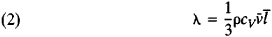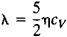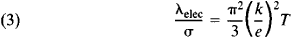# Thermal Conduction

Also found in: Dictionary, Thesaurus, Medical, Wikipedia.
Related to Thermal Conduction: Heat transfer

## Thermal conduction

The process of heat transfer through a material medium, in which kinetic energy is transmitted by particles of the material without displacement of the particles.
The following article is from The Great Soviet Encyclopedia (1979). It might be outdated or ideologically biased.

## Thermal Conduction

(or heat conduction), one of the ways in which heat, that is, the energy of thermal motion of mi-croparticles, is transferred from hotter regions of a substance to less hot regions so as to equalize the temperatures of the regions.

Thermal conduction involves the transfer of energy directly from particles—such as molecules, atoms, or electrons—with high energies to particles with lower energies. If the relative change in temperature T at the distance of the mean free path Ī of a particle is small, then the Fourier law holds: the heat flux q is proportional to the temperature gradient grad T —that is,

(1) q = –λ gred T

Here, λ is the coefficient of thermal conductivity, or simply the thermal, or heat, conductivity. It is independent of grad T and depends on such factors as the state of aggregation of the substance (see Table 1), the atomic and molecular structure of the substance, the temperature, the pressure, and, in the case of a mixture or solution, the composition of the substance.

Table 1. Thermal conductivity λ of some gases, liquids, and solids at atmospheric pressure
SubstanceTemperature (°C)λ (watts/m°K)
Gases
Hydrogen ...............00.1655
Helium ...............00.1411
Oxygen ...............00.0239
Nitrogen ...............–30.0237
Air ...............40.0226
Metals
Silver ...............0429
Copper ...............0403
Iron ...............086.5
Tin ...............068.2
Liquids
Mercury ...............07.82
Water ...............200.599
Acetone ...............160.190
Ethyl alcohol ...............200.167
Benzene ...............22.50.158
Minerals and materials
Sodium chloride ...............06.9
Tourmaline ...............04.6
Glass ...............180.4–1
Wood ...............180.16–0.25
Asbestos180.12

Deviations from the Fourier law may occur in the following cases: at high values of grad T, for example, in intense shock waves; at low temperature (for liquid helium He II); and at high temperatures of the order of tens or hundreds of thousands of degrees. In gases at such high temperatures, energy is transferred predominantly not through collisions between atoms but through radiation. In dilute gases in which Ī is comparable with the distance L between the walls bounding the volume of gas, the molecules collide more frequently with the walls than with each other. The conditions for the applicability of the Fourier law are violated here, and the very concept of a local gas temperature becomes meaningless. In this case, heat transfer between bodies in the gaseous medium is considered, rather than the process of thermal conduction in the gas. Conductive heat transfer in a continuous medium is described by the heat equation.

For an ideal gas consisting of rigid spherical molecules of diameter d, the following expression holds, according to the kinetic theory of gases, for λ when d « l « L:where ρ is the density of the gas, cv is the specific heat of the gas at constant volume V, and v is the mean velocity of the molecules. Since Ī is proportional to 1/p and ρ ~ p, where ρ is the gas pressure, the thermal conductivity of such a gas does not depend on the pressure. Moreover, λ and the viscosity η are related by the following equation:In the case of a gas consisting of polyatomic molecules, an important contribution to λ is made by the internal degrees of freedom of the molecules. This fact is taken into account by the equation

λ = ηcv[(9γ – 5)/4]

where γ = cp/cv (c p is the specific heat at constant pressure). In the case of real gases, the thermal conductivity is a rather complicated function of temperature and pressure and increases with increasing T and p. The value of λ for a gaseous mixture may be larger or smaller than that for the components of the mixture—that is, the thermal conductivity is a nonlinear function of composition.

In dense gases and liquids, the mean distance between the molecules is comparable with the dimensions of the molecules, and the kinetic energy of the molecules is of the same order as the intermolecular potential. Thus, the transfer of energy through collisions is much more intense than in dilute gases, and the rate of transfer of molecular energy from hot isothermal fluid layers to cooler layers is close to the rate of propagation of small pressure disturbances. Since this rate of propagation is equal to the speed of sound, we have λ = ρcpus L̄ where us is the speed of sound in the fluid and L̄ is the mean distance between the molecules. This equation is best satisfied by monatomic liquids. The value of λ for liquids generally decreases with increasing T and slowly increases with increasing p.

The nature of thermal conduction in solids depends on the type of solid. In dielectrics, which lack free electric charges, the energy of thermal motion is transported by the quasiparticles known as phonons, which are quanta of the elastic vibrations of the atoms of a crystal (seeLATTICE_VIBRATIONS and QUASIPARTICLES). In solid dielectrics, λ ≈ cv̄l̄. Here, c is the specific heat of the dielectric, which is the same as the specific heat of the phonon gas; is the mean velocity of the phonons, which is approximately equal to the speed of sound, and is the mean free path for the phonons. The existence of a definite finite value of is a consequence of the scattering of phonons by phonons and by lattice imperfections, particularly grain boundaries and the boundary of the specimen. The temperature dependence of λ is determined by the temperature dependence of c and . At high temperatures (T » θD, where θD is the Debye temperature), the phonon-phonon scattering associated with the anharmonicity of the vibrations of the atoms in the crystal is the principal mechanism limiting . The phonon-phonon mechanism of thermal resistance (1/λ is known as the thermal resistivity) is accounted for by Umklapp processes, as a result of which the phonon flux is slowed down. The greater the value of T, the lower the value of and the greater the probability of the occurrence of Umklapp processes. When T » θD, Ī ~ 1/T, and, consequently, λ ~ 1/T, since under these conditions c depends weakly on T. As T decreases (T « θD), the mean free path determined by phonon-phonon scattering markedly increases [ ~ exp (θD /T)] and is generally limited by the size R of the specimen. When T « θD, the specific heat decreases approximately as T3. Consequently, λ passes through a maximum with decreasing temperature. The temperature at which maximum λ occurs is determined by the approximate equality (T) ≈ R.

In metals, thermal conduction is a result primarily of the motion and interaction of current carriers, that is, conduction electrons. In the general case, the thermal conductivity of a metal is equal to the sum of a lattice phonon component λlat, and an electronic component λelec: λ = λlat + λelec. Moreover, at ordinary temperatures generally λelec » λlat. In the thermal conduction process, each electron transports, when a temperature gradient is present, the quantity of energy kT, where k is the Boltzmann constant. Consequently, the Wiedemann-Franz law holds over a broad range of temperatures. It states that the ratio of the electronic component λelec of the thermal conductivity to the electrical conductivity a is proportional to the temperature:where e is the electronic charge. Since in most metals λlat « λelec, λelec can be replaced by λ in the Wiedemann-Franz law with a high degree of accuracy. Observed deviations from (3) have been explained by the inelasticity of electron collisions. In the case of the semimetals Bi and Sb, λlat is comparable with λdec because of the small number of free electrons.

The phenomenon of heat transfer in semiconductors is more complicated than in dielectrics and metals for two reasons: first, both components of the thermal conductivity, λelec and λlat, are important in semiconductors; second, the thermal conductivity of semiconductors is greatly affected by impurities, bipolar diffusion processes, exciton transfer, and other factors.

The influence of pressure on the λ of solids is expressed with good accuracy by a linear dependence of λ on p. In many metals and minerals, λ increases with increasing. p

### REFERENCES

Lykov, A. V. Teoriia teploprovodnosti. Moscow, 1967.
Reif, F. Statisticheskaia fizika. (Berkleevskii kurs fiziki, vol. 5.) Moscow, 1972. (Translated from English.)
Roberts, J. Teplota i termodinamika. Moscow-Leningrad, 1950. (Translated from English.)
Hirschfelder, J., C. Curtiss, and R. Bird. Molekuliarnaia teoriia gazov izhidkostei. Moscow, 1961. (Translated from English.)
Ziman, J. Printsipy teorii tverdogo tela. Moscow, 1966. (Translated from English.)
Kittel, C. Elementarnaia fizika tverdogo tela. Moscow, 1965. (Translated from English.)
Zel’dovich, Ia. B., and Iu. P. Raizer. Fizika udarnykh voln i vysoko-temperatumykh gidrodinamicheskikh iavlenii, 2nd ed. Moscow, 1966.

S. P. MALYSHENKO

## thermal conduction

The process of heat transfer through a material medium in which kinetic energy is transmitted by particles of the material from particle to particle without gross displacement of the particles.
McGraw-Hill Dictionary of Architecture and Construction. Copyright © 2003 by McGraw-Hill Companies, Inc.
References in periodicals archive ?
In this manner, thermal conduction can occur between the two cavities, if necessary.
where [rho] is density, C is specific heat, T is temperature of medium, t is time, [??] is a tensor representing thermal conduction coefficient, and Q is internal heat generated per volume.
The term [nabla] x ([kappa] x [nabla]T) represents the thermal conduction. The symbol L is heat-loss function and is given by
The mechanism of thermal conduction and electrical conduction is different in the epoxy nanocomposites with MWNT loading.
Addressing the increasing demand for automated washroom functionality driven by sensor-based convenience systems, GlobTek's Level V GT-41076-0609-2.5-IS-P2 family of inline encapsulated power supplies has IP64 ingress protection and is potted in a 75 mm x 35 mm x 24.6 mm impact-resistant polycarbonate case with thermal conduction cooling.
Materials should have small thermal conduction and adequate strength characteristics in order to be used in building enclosures in which different short-term and long-term loads act and which could compete with nonecological contemporary effective thermal insulations.
- Evaluation of thermal diffusion and thermal conduction of materials around FPD
Thermal conduction by minerals is of little importance compared to the thermal convection of groundwater moving through the pores of the rocks.
Because thermoelectric materials have a finite electric resistivity and a finite thermal conductivity, these devices are subject to two primary losses, electric resistance loss and thermal conduction, that increase the power input and reduce the net flow of heat from cold to hot.
Thermal models for investigation may be divided into two main categories: models with detailed treatment of thermal conduction and models where details of phase transition (melting, vaporization) are considered.
PP FlexEdge can accommodate tight radii while delivering chemical resistance, light fastness, shrinkage and thermal conduction properties that are competitive with traditional PVC materials, REHAU says.
which promotes their thermal conduction. Rapid heterogeneous nucleation occurs at the cooler boundaries of the flattened droplets in a large undercooling.

Site: Follow: Share:
Open / Close